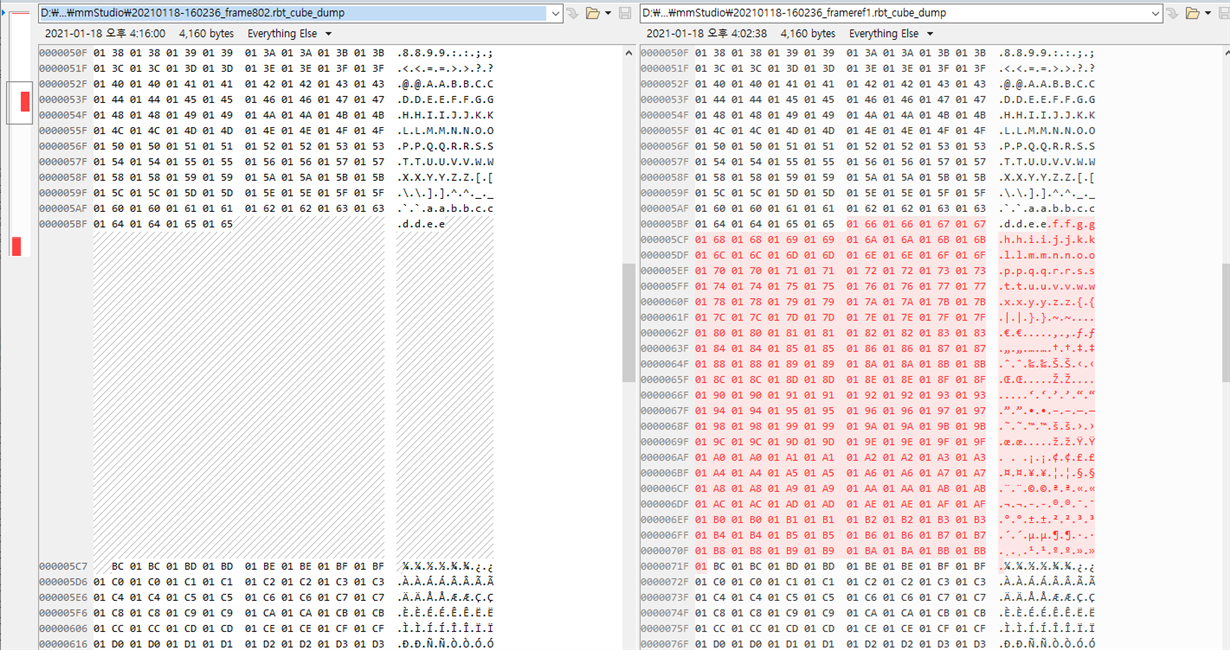# IWR6843ISK: IWR6843ISK missing data while reading it on "mmWave_Demo_Visualizer"

Part Number: IWR6843ISK

Dear TI.

I have a problem missing uart data.
I modified mss_main.c to send data of 4KB size which was filled indexes, and also I mofied "mmWave_Demo_Visualizer" to receive and to check data if it miss the data.

I have tested with 2 iwr6843isk evm board. both of them have same issue.

i have tesed it on windows 10 with installed l"mmWave_Demo_Visualizer".

let me know how i can fix it.

belows are modified sources and log:

logs:
mmWave.js:3430 processCubeData error: idx=444 imag=51457 real=51457
mmWave.js:3430 processCubeData error: idx=445 imag=51713 real=51713
mmWave.js:3430 processCubeData error: idx=446 imag=51969 real=51969
mmWave.js:3430 processCubeData error: idx=447 imag=52225 real=52225
mmWave.js:3430 processCubeData error: idx=448 imag=52481 real=52481
mmWave.js:3430 processCubeData error: idx=449 imag=52737 real=52737

mmWave.js :

} else if (tlvtype == TLV_type.MMWDEMO_OUTPUT_MSG_TEMPERATURE_STATS + 1) { // JACK
processCubeData(bytevec, byteVecIdx, tlvlength, Params);
}
byteVecIdx += tlvlength;

..

var processCubeData = function (bytevec, byteVecIdx, tlvlength, Params) {
var idx;
var arr;
var imag = 0;
var real = 0;
var error = 0;

var dataLength = tlvlength / 4;

if (error == 0) {
}
for (idx = 0; idx < dataLength; idx++) {
arr = bytevec.slice(byteVecIdx, byteVecIdx + 4);
byteVecIdx += 4;

imag = arr + (arr << 8);
real = arr + (arr << 8);

if (idx != imag || idx != real) {
console.log('processCubeData error:' + ' idx=' + idx + ' imag=' + imag + ' real=' + real);
error = 1;
}
}

if (error == 1) {
}

}

### 7 Replies

• Dear TI.

evm source is based on mmwave_sdk_68xx_dsp_mss of out_of_box_demo.

it send 4KB on MmwDemo_transmitProcessedOutput.

tl[tlvIdx].type = MMWDEMO_OUTPUT_MSG_TEMPERATURE_STATS + 1; //MMWDEMO_OUTPUT_MSG_RADARCUBE_DATA;
tl[tlvIdx].length = gRadarcube_size * sizeof(cmplx16ImRe_t); // 1KByte
packetLen += sizeof(MmwDemo_output_message_tl) + tl[tlvIdx].length;
tlvIdx++;

UART_writePolling (uartHandle,
(uint8_t*)&tl[tlvIdx],
sizeof(MmwDemo_output_message_tl));

thanks.

• In reply to Hoyeon JANG:

HI Jang,

Is it failing for all the frames or only the last one.

Thanks

Yogesh

• In reply to Yogesh Siraswar:

Hi Yogesh,

some frames are success, and some frames are failed.

logs:
mmWave.js:3419 processCubeData: Params.frameNumber 2064                           <- success
mmWave.js:3419 processCubeData: Params.frameNumber 2065                           <- success
mmWave.js:3430 processCubeData error: idx=1020 imag=65027 real=65283
mmWave.js:3430 processCubeData error: idx=1021 imag=65283 real=62467
mmWave.js:3430 processCubeData error: idx=1022 imag=227 real=264
mmWave.js:3430 processCubeData error: idx=1023 imag=0 real=14336
mmWave.js:3436 processCubeData error: Params.frameNumber 2066                    <- failed
mmWave.js:3419 processCubeData: Params.frameNumber 2068                             <- success

.......

mmWave.js:3419 processCubeData: Params.frameNumber 3035                            <- success
mmWave.js:3419 processCubeData: Params.frameNumber 3036                            <- failed
mmWave.js:3430 processCubeData error: idx=284 imag=7681 real=7937
mmWave.js:3430 processCubeData error: idx=285 imag=7937 real=8193
mmWave.js:3430 processCubeData error: idx=286 imag=8193 real=8449
....
mmWave.js:3430 processCubeData error: idx=1020 imag=65027 real=65283
mmWave.js:3430 processCubeData error: idx=1021 imag=65283 real=62467
mmWave.js:3430 processCubeData error: idx=1022 imag=227 real=264
mmWave.js:3430 processCubeData error: idx=1023 imag=0 real=14336
mmWave.js:3436 processCubeData error: Params.frameNumber 3036                  <- failed
mmWave.js:3419 processCubeData: Params.frameNumber 3038                           <- success

thanks.

• In reply to Hoyeon JANG:

HI,

Please check if you have the latest firmware of XDS110. There were packets drops seen with older version. Please refer to following thread:

https://e2e.ti.com/support/tools/ccs/f/81/p/966457/3578265

Thanks

Yogesh

• In reply to Yogesh Siraswar:

Dear Yogesh,

i would check xds110,

but I tested with only iwr6843isk, without mmwaveicboost.

iwr6843isk has silicon labs dual cp2105

thanks,

hoyeon.

• In reply to Hoyeon JANG:

Dear Yogesh,

i have checked with xds110 of mmwaveicboost.

there is still problem missing data. (i think using xds110 is less than iwr6843isk only)

following image shows that it was missing data at 802th-frame compare to 1st-frame.xds110's firmware is 3.0.0.14.

thanks,

hoyeon.

• In reply to Hoyeon JANG:

Hi Hoyeon,

Did you try and increase the frame size to see the data is not being truncated.

Thanks

Yogesh